# Write short notes on , RC Oscillator , Wien bridge Oscillator & Crystal Oscillator

## RC Oscillator

### Principle of RC Oscillator Or Phase Shift Oscillator

Good frequency stability and waveform can be obtained from oscillators employing resistive and capacitive elements. Such oscillators are called R-C or phase shift oscillators.

These oscillators have additional advantage of being used at a very low frequencies.

In a phase shift oscillator, a phase shift of 180is obtained with a phase shift circuit instead of inductive or capacitive coupling.

A further phase shift of 180is introduced due to the transistor properties. Thus energy supplied back to the tank circuit is of correct phase.

Phase shift Circuit:

A phase shift circuit consists of an R-C network. Fig.1 (i) shows a single section of RC network.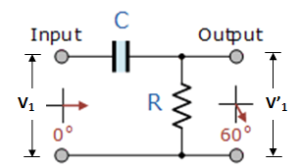Fig.1 (i)

As we know the alternating voltage V’1 across R leads the applied voltage Vby Φ0. The value of Φ depends upon the value of R and C. If resistance R is varied, the value of Φ also changes.

If R were reduced to zero, V’will lead  Vby 90i.e Φ=900.

However, adjusting R to zero would be impracticable because it would lead to no voltage across R. Therefore, in practice, R is varied to such a value that makes V’1 to lead V1 by 600 .

Fig.1 (ii) shows the three sections of RC network. Each section produces a phase shift of 600. Consequently, a total phase shift of 180is produced.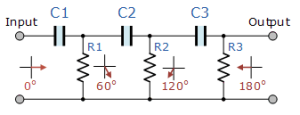Fig.1 (ii)

### Phase shift Oscillator

Fig.2 shows the circuit of a phase shift oscillator.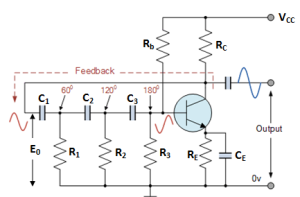Fig.2

It consists of a conventional single transistor amplifier and a RC phase shift network.

The phase shift network consists of three sections of R1C1  , R2Cand R3C3.

At some particular frequency f0, the phase shift in each RC section is 60so that the total phase shift produced by the RC network is 1800.

The frequency of oscillation is given by:

Where,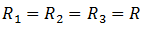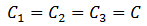#### Circuit Operation:

When the circuit is switched on, it produces oscillations of frequency determined by exp.(i) .

The output Eof the amplifier is fed back to RC feedback network. This network produces a phase shift of 1800 and a voltage Eappears at its output which is applied to the transistor amplifier.

The feedback fraction m =E/ E0.

The phase shift of 180is produced by the transistor amplifier and a further phase shift of 180is produced by the RC network. As a result, the phase shift around the entire loop is 3600.

1. It does not require transformers or inductors.
2. It can be used to produce very low frequencies.
3. The circuit provides good frequency stability.

1. It is difficult for the circuit to start oscillations as the feedback is generally small.
2. The circuit gives small output.

## Wien Bridge Oscillator

The wien-bridge oscillator is the standard oscillator circuit for all frequencies in the range of 10 Hz to about 1 MHz.

It is the most widely frequently used type of audio oscillator as the output is free from circuit fluctuations and ambient temperature.

Fig.3 shows the circuit of a wien-bridge oscillator.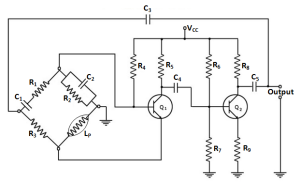Fig.3

It is essentially a two stage amplifier with R-C bridge circuit.

The bridge circuit has the arms R1C1 , R3, R2C2 and tungsten lamp Lp.

Resistances Rand Lare used to stabilise the amplitude of the output.

The transistor Tserves as an oscillator and amplifier while the other transistor Tserves as an inverter to produce 1800 phase shift.

The circuit uses positive and negative feedback. The positive feedback is through R1C1 , C2R2 to the transistor T1. The negative feedback is through the voltage divider to the input of transistor T2.

The frequency of oscillation is determined by the series element R1C1 and parallel element C2Rof  the bridge.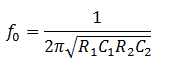If ,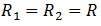and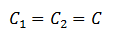then,

#### Circuit Action

When the circuit is started, bridge circuit produces oscillations of frequency determined by exp.(i).

The two transistors produce a total phase shift of 360so that proper positive feedback is ensured.

The negative feedback in the circuit ensures constant output. This is achieved by the temperature sensitive tungsten lamp Lp. Its resistance increases with current. So when the amplitude of the output tend to increase, more current would provide more negative feedback. The result is that the output would return to original value.  A reverse action would take place if the output tends to decrease.

### Advantages of Wien Bridge Oscillator

1. It gives constant output.
2. The circuit works quite easily.
3. The overall gain is high because of two transistors.
4. The frequency of oscillations can be easily changed by using potentiometer.

### Disadvantages of Wien Bridge Oscillator

1. The circuit requires two transistors and a large number of components.
2. It can not generate very high frequencies

## Quartz Crystal Oscillator

Quartz crystals are generally used in crystal oscillators because of their great mechanical strength and simplicity in manufacture.

The natural frequency f  of a crystal is given by: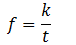Where K = a constant that depends upon the cut

t = the thickness of the crystal

In order to use crystal in an electronic circuit, it is placed between two metal plates. The arrangement then forms a capacitor with crystal as the dielectric as shown in fig.4.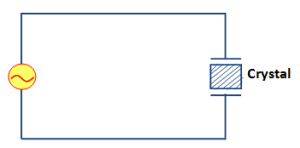Fig.4

If an a.c. voltage is applied across the plates, the crystal will start vibrating at the frequency of applied voltage.

However, if the frequency of the applied voltage is made equal to the natural frequency of the crystal , resonance takes place and crystal vibration reach a maximum value. This natural frequency is almost constant.

### Equivalent Circuit of Crystal Oscillator

When the crystal is not vibrating, it is equivalent to capacitance Cm because it has two metal plates separated by a dielectric as shown in fig.5(i). This capacitance is known as mounting capacitance.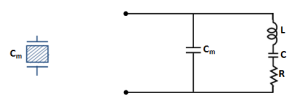Fig.5 (i)                                          Fig.5 (ii)

When a crystal vibrates, it is equivalent to R-L-C- series circuit.

Therefore, the equivalent circuit of a vibrating crystal is R-L-C series circuit shunted by the mounting capacitance Cas shown in fig.5 (ii).

## Transistor Crystal Oscillator

Fig.6 shows the transistor crystal oscillator.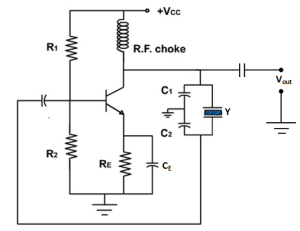Fig.6

It is a Collpit’s oscillator modified to act as a crystal oscillator. Th only change is the addition of the crystal (Y) in the feedback network.

The crystal will act as a parallel-tuned circuit.

As we can see in this circuit that instead of resonance caused by L and  (C1+C2), we have the parallel resonance of the crystal.

At parallel resonance, the impedance of the crystal is maximum. This means that there is a maximum voltage drop across C1. This in turn will allow the maximum energy transfer through the feedback network at  the parallel resonant frequency fp which is given by:Where,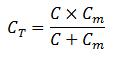Note that feedback is positive. A phase shift of is produced by the transistor. A further phase shift of is produced by the capacitor voltage divider.

This oscillator will oscillate only at fp . Even a small deviation from fwill cause the oscillator to act as an effective short. Consequently, we have an extremely stable oscillator.